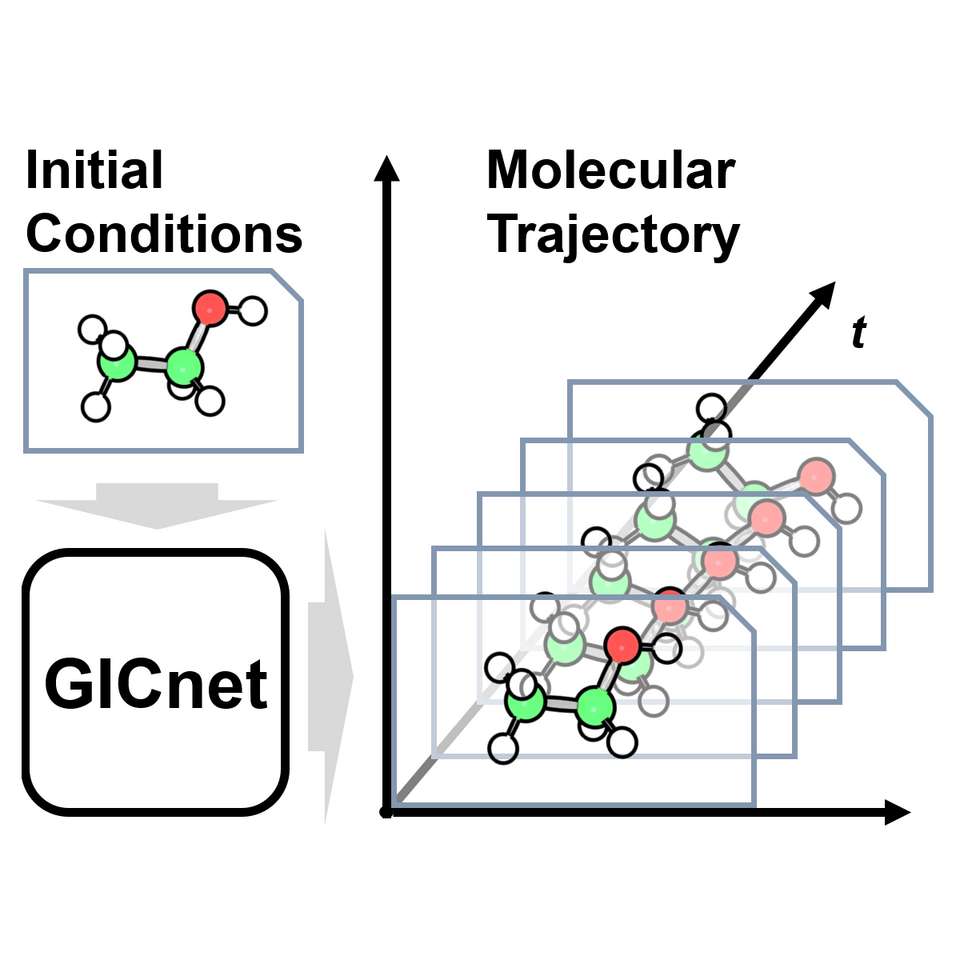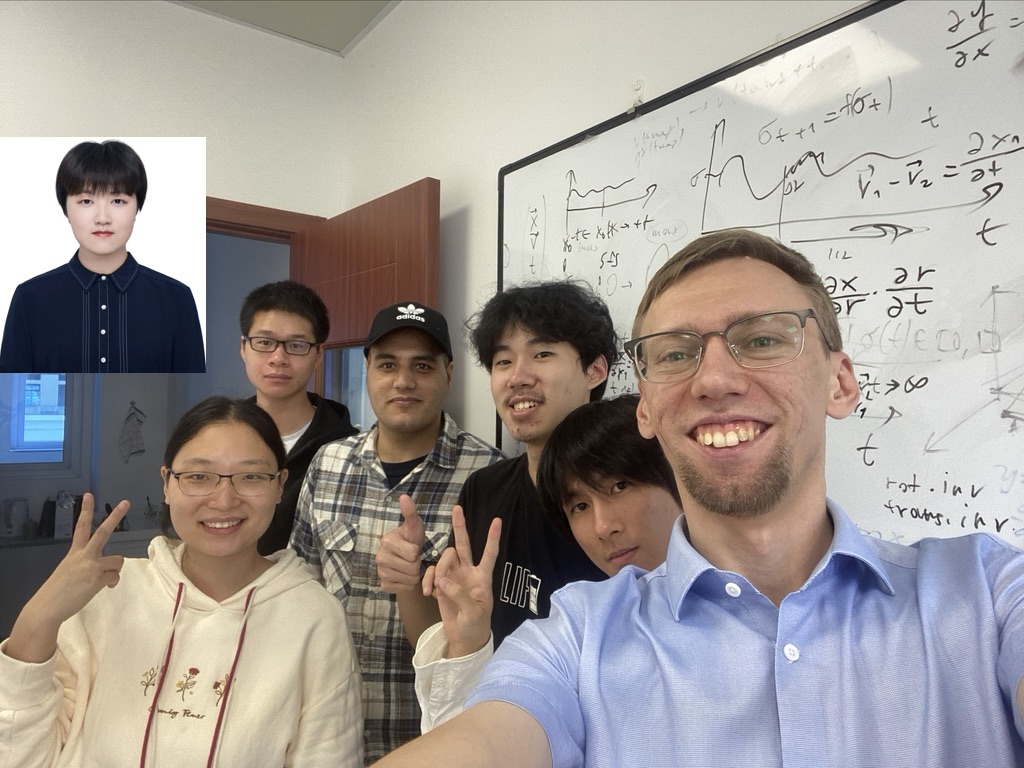# Beyond 3D-Machine Learning Interatomic Potentials: Meet 4D-Spacetime Atomistic Artificial Intelligence Models

We have introduced a concept of 4D-spacetime atomistic AI models that learn how the molecule changes in timeWe have introduced a concept of 4D-spacetime atomistic AI models that learn how the molecule changes in time. We demonstrate that this concept is feasible by developing the 4D-spacetime GICnet models that directly predict the atomic coordinates of a molecule as a continuous function of time. It is a radically different alternative to traditional molecular dynamics that predicts nuclear coordinates with slow consecutive, one-step-at-a-time, algorithms at discrete time steps.

The molecules are always in motion and it is highly desirable to simulate their time-dependent, dynamic, behavior. Unfortunately, accurate dynamics is very resource-consuming endeavor and it is often done with many approximations. One of the most widely used approximations is molecular dynamics which calculates how much atoms are moved in a very small time step and then repeats this procedure many times until the required time is reached. Each of these steps requires the evaluation of forces acting on atoms. The problem is that calculating forces is not a trivial task but it is now often accelerated with so-called machine learning interatomic potentials. Nevertheless, such ML approaches still do not obliterate the need of running slow sequential algorithms with discrete time step.

Advances in AI for science allow us to look at the problem from a radically new angle: why not predict the atomic positions for the required time directly with AI? This was an idea floating around in our group for quite some time and, back then, we had been working on a paper on the related AI-quantum dynamics approach that also learns quantum dynamics trajectories as a continuous function of time. We finally set to work on learning atomic positions as a function of time in our meeting on October 29, 2021.29 October 2021, when we set to work on the 4D-spacetime AI models. From right to left: Pavlo, Fuchun (the main developer of 4D-GICnet models), Yi-Fan (developer of traditional MD code, IR and power spectra simulations), Arif (developer of the related AI-quantum dynamics approach), Peikun (developer of the AI-quantum mechanical method 1), Lina (main user and tester of ground-state dynamics approaches and a specialist in nonadiabatic dynamics). Xinxin joined the group later and contributed with extracting insight from the 4D-GICnet models for a reaction (her research interest is application of ML to reactions).

We could show that for simplest systems like diatomics, the realization of the concept is rather straightforward and ML basically can predict the configuration at any time. However, for polyatomic molecules such as ethanol or azobenzene, the dimensionality curse kicks. For such molecular systems, we developed more general GICnet model that is able to predict the nuclear coordinates up to rather distant time: 10-20 fs. This time is much longer than typical time steps used in molecular dynamics (e.g., preferably below 1 fs).

One can use the GICnet models to obtain molecular trajectories much faster than with traditional MD algorithms (even accelerated with machine learning interatomic potentials) and we can parallelize predictions at different time steps as they do not need to be calculated sequentially. The trajectories also can be obtained with arbitrarily high resolution. This allows to also calculate fast the properties which can be derived from MD trajectories and we demonstrate this by generating vibrational spectra.

The GICnet models are of course much more than simply faster MD algorithm. The neural networks condense the information about a molecule in 4D spacetime. This can be used, e.g., to get insights into the coupled nuclear motions whose interplay are otherwise difficult to interpret. For the demonstration, we analyzed how different coordinates affect the cis-trans isomerization of azobenzene.

The future will surely see the shift of dynamics simulations from traditional MD to 4D-spacetime AI models. To reduce the entry barrier, we provide our models in open-source MLatom and the simulations can be also run on MLatom@XACS cloud.

Paper: